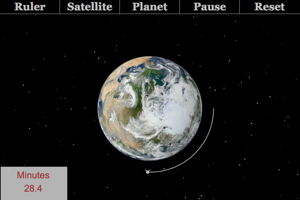Information About The Program
Satellite Playground (Lab)This lab is designed to allow students to explore different aspects of satellite motion. Students can vary the mass of the satellite, the orbital radius of the satellite and the body that the satellite is orbiting. Students can measure the time of an orbit and then calculate speed, orbits per day, acceleration or kinetic energy.
Below are any Resources that go with this program